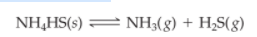×
Get Full Access to Introductory Chemistry - 5 Edition - Chapter 15 - Problem 104p
Get Full Access to Introductory Chemistry - 5 Edition - Chapter 15 - Problem 104p

×

# Solved: Consider the reaction: A sample of pure NH4HS is placed in a sealed 2.0-LISBN: 9780321910295 34

## Solution for problem 104P Chapter 15

Introductory Chemistry | 5th Edition

• Textbook Solutions
• 2901 Step-by-step solutions solved by professors and subject experts
• Get 24/7 help from StudySoup virtual teaching assistantsIntroductory Chemistry | 5th Edition

4 5 1 387 Reviews
27
2
Problem 104P

Consider the reaction:

$$\mathrm{NH}_{4} \mathrm{HS}(s) \rightleftharpoons \mathrm{NH}_{3}(g)+\mathrm{H}_{2} \mathrm{~S}(g)$$

A sample of pure $$\mathrm{NH}_{4} \mathrm{HS}$$ is placed in a sealed 2.0-L container and heated to 550 K at which the equilibrium constant is $$3.5^{*} 10^{-3}$$. Once the reaction reaches equilibrium, what mass of $$\mathrm{NH}_{3}$$ is in the container? (Assume that the sample of $$\mathrm{NH}_{4} \mathrm{HS}$$ was large enough that equilibrium could be achieved.)

Equation Transcription:NH4HS

3.5 * 10-3

NH3

Text Transcription:

NH_4HS(s) harpoon NH_3(g) + H_2S(g)

NH_4HS

3.5 times 10^-3

NH_3

Step-by-Step Solution:

Solution 104P:

Step 1:The equilibrium constant is

Keq == [NH3][H2S]

Thus, we know that, NH4HS(s) is a solid, so that it is not included into the equilibrium constant.

Keq= 3.5 × 10‒3.

[NH3][H2S] =  3.5 × 10‒3.

From equation, 1 mol NH4HS(s) produces 1.0 mol of NH3(g) and (H2S each.

Thus,

[NH3] = [H2S]

Therefore.

Keq =  [NH3][H2S] =  [NH3]2[NH3]2 = 3.5 × 10‒3.[NH3] == 0.059 M

Step 2 of 3

Step 3 of 3

##### ISBN: 9780321910295

Since the solution to 104P from 15 chapter was answered, more than 490 students have viewed the full step-by-step answer. This full solution covers the following key subjects: Equilibrium, reaction, sample, container, large. This expansive textbook survival guide covers 19 chapters, and 2046 solutions. The full step-by-step solution to problem: 104P from chapter: 15 was answered by , our top Chemistry solution expert on 05/06/17, 06:45PM. Introductory Chemistry was written by and is associated to the ISBN: 9780321910295. This textbook survival guide was created for the textbook: Introductory Chemistry, edition: 5. The answer to “?Consider the reaction:$$\mathrm{NH}_{4} \mathrm{HS}(s) \rightleftharpoons \mathrm{NH}_{3}(g)+\mathrm{H}_{2} \mathrm{~S}(g)$$A sample of pure $$\mathrm{NH}_{4} \mathrm{HS}$$ is placed in a sealed 2.0-L container and heated to 550 K at which the equilibrium constant is $$3.5^{*} 10^{-3}$$. Once the reaction reaches equilibrium, what mass of $$\mathrm{NH}_{3}$$ is in the container? (Assume that the sample of $$\mathrm{NH}_{4} \mathrm{HS}$$ was large enough that equilibrium could be achieved.)Equation Transcription: NH4HS3.5 * 10-3NH3Text Transcription:NH_4HS(s) harpoon NH_3(g) + H_2S(g)NH_4HS3.5 times 10^-3NH_3” is broken down into a number of easy to follow steps, and 71 words.

Unlock Textbook Solution